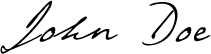# О нас

Lorem ipsum dolor sit amet, consectetur adipisicing elit. At pariatur officiis reprehenderit, aperiam tempora sint iusto optio omnis, eaque officia, unde incidunt accusantium animi itaque quibusdam magni soluta labore qui. Sunt fuga consectetur officia tenetur minus recusandae, facilis, totam ab illum velit ex sit nemo excepturi quo. Nobis cupiditate magnam, fugiat dignissimos architecto odio enim numquam quo harum quaerat eum ipsum est et molestiae modi ullam odit fuga nam explicabo dolores rerum reprehenderit. Velit, impedit incidunt voluptate praesentium vel odio.## Who we are

In non-Euclidean geometry, squares are more generally polygons with 4 equal sides and equal angles.In spherical geometry, a square is a polygon whose edges are great circle arcs of equal distance, which meet at equal angles. Unlike the square of plane geometry, the angles of such a square are larger than a right angle. Larger spherical squares have larger angles. In hyperbolic geometry, squares with right angles do not exist. Rather, squares in hyperbolic geometry have angles of less than right angles. Larger hyperbolic squares have smaller angles. Squares can tile the hyperbolic plane with 5 around each vertex, with each square having 72-degree internal angles. Six squares can tile the sphere with 3 squares around each vertex and 120-degree internal angles. This is called a spherical cube.

## What we do

### WEB DESIGN

In hyperbolic geometry, squares with right angles do not exist. Rather, squares in hyperbolic geometry have angles of less than right angles.

### PRINT DESIGN

In hyperbolic geometry, squares with right angles do not exist. Rather, squares in hyperbolic geometry have angles of less than right angles.

### PHOTOGRAPHY

In hyperbolic geometry, squares with right angles do not exist. Rather, squares in hyperbolic geometry have angles of less than right angles.

### MARKETING & PR

In hyperbolic geometry, squares with right angles do not exist. Rather, squares in hyperbolic geometry have angles of less than right angles.

## Our team

RICHARD JONES CO-FOUNDER

In non-Euclidean geometry, squares are more generally polygons with 4 equal sides and equal angles.

DIANA KRUGER FINANCIAL DIRECTOR

In non-Euclidean geometry, squares are more generally polygons with 4 equal sides and equal angles.

ARTHUR CLARK CO-FOUNDER

In non-Euclidean geometry, squares are more generally polygons with 4 equal sides and equal angles.

EMMY JHONSON ART-DIRECTOR

In non-Euclidean geometry, squares are more generally polygons with 4 equal sides and equal angles.

## Our skills

### Web Design

Six squares can tile the sphere with 3 squares around each vertex and 120-degree internal angles. This is called a spherical cube.

### Print Design

Six squares can tile the sphere with 3 squares around each vertex and 120-degree internal angles. This is called a spherical cube.

### Photography

Six squares can tile the sphere with 3 squares around each vertex and 120-degree internal angles. This is called a spherical cube.

### MARKETING & PR

Six squares can tile the sphere with 3 squares around each vertex and 120-degree internal angles. This is called a spherical cube.